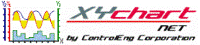XY Chart NET 3 Control Reference
MaxYValue Property

Use this property to obtain the current maximum y-value, of a specific y-axis, displayed on the graph.  The value returned is of type Double.

Syntax
 [Visual Basic] ReadOnly Property MaxYValue(YAxisNumber As Integer) As Double [C#] double XYChartNETCtl.get_MaxYValue(int YAxisNumber) [C++] property double MaxYValue[int]

Parameters
YAxisNumber
The y-axis index to obtain the current maximum value of.  Valid values are 0..(NumYScales - 1).
Remarks

This property can be used to obtain the current maximum y-value displayed on the graph after a zoom-in has occurred.

Example
```' XYChartNETCtl1 is the name of the XY Chart NET control instance placed on the form.

Dim idx As Integer
Dim tmpMin As Double
Dim tmpMax As Double

For idx = 0 To XYChartNETCtl1.NumYScales - 1
tmpMin = XYChartNETCtl1.MinYValue(idx)  'Get the current min value for the specified y-axis
tmpMax = XYChartNETCtl1.MaxYValue(idx)  'Get the current max value for the specified y-axis
Next idx
```
```// XYChartNETCtl1 is the name of the XY Chart NET control instance placed on the form.

double tmpMin;
double tmpMax;

tmpMin = XYChartNETCtl1.get_MinYValue(1);  //Get the current min value for y-axis 1
tmpMax = XYChartNETCtl1.get_MaxYValue(1);  //Get the current max value for y-axis 1
```
```// XYChartNETCtl1 is the name of the XY Chart NET control instance placed on the form.

double tmpMin;
double tmpMax;

tmpMin = XYChartNETCtl1->MinYValue;  //Get the current min value for y-axis 0
tmpMax = XYChartNETCtl1->MaxYValue;  //Get the current max value for y-axis 0
```